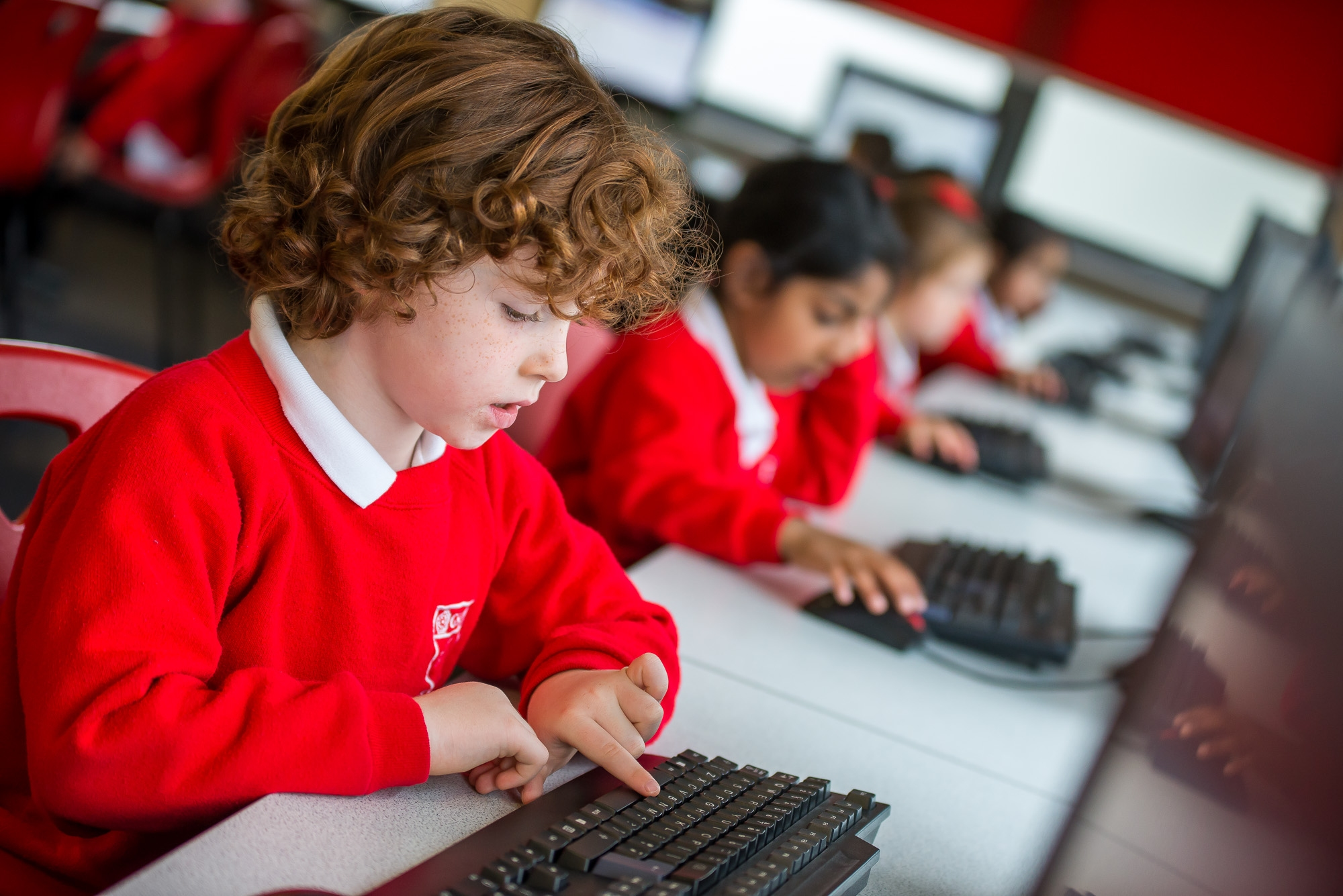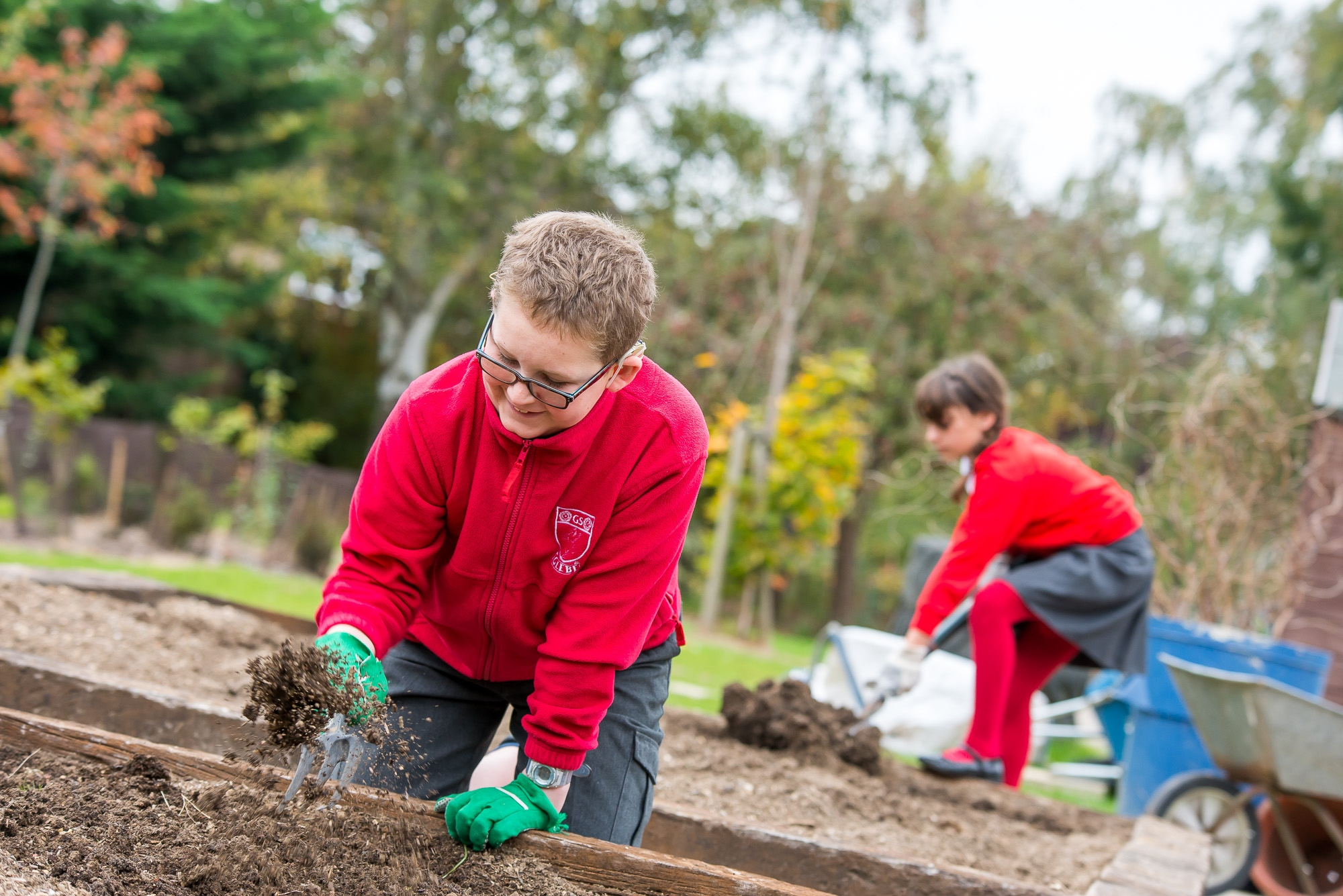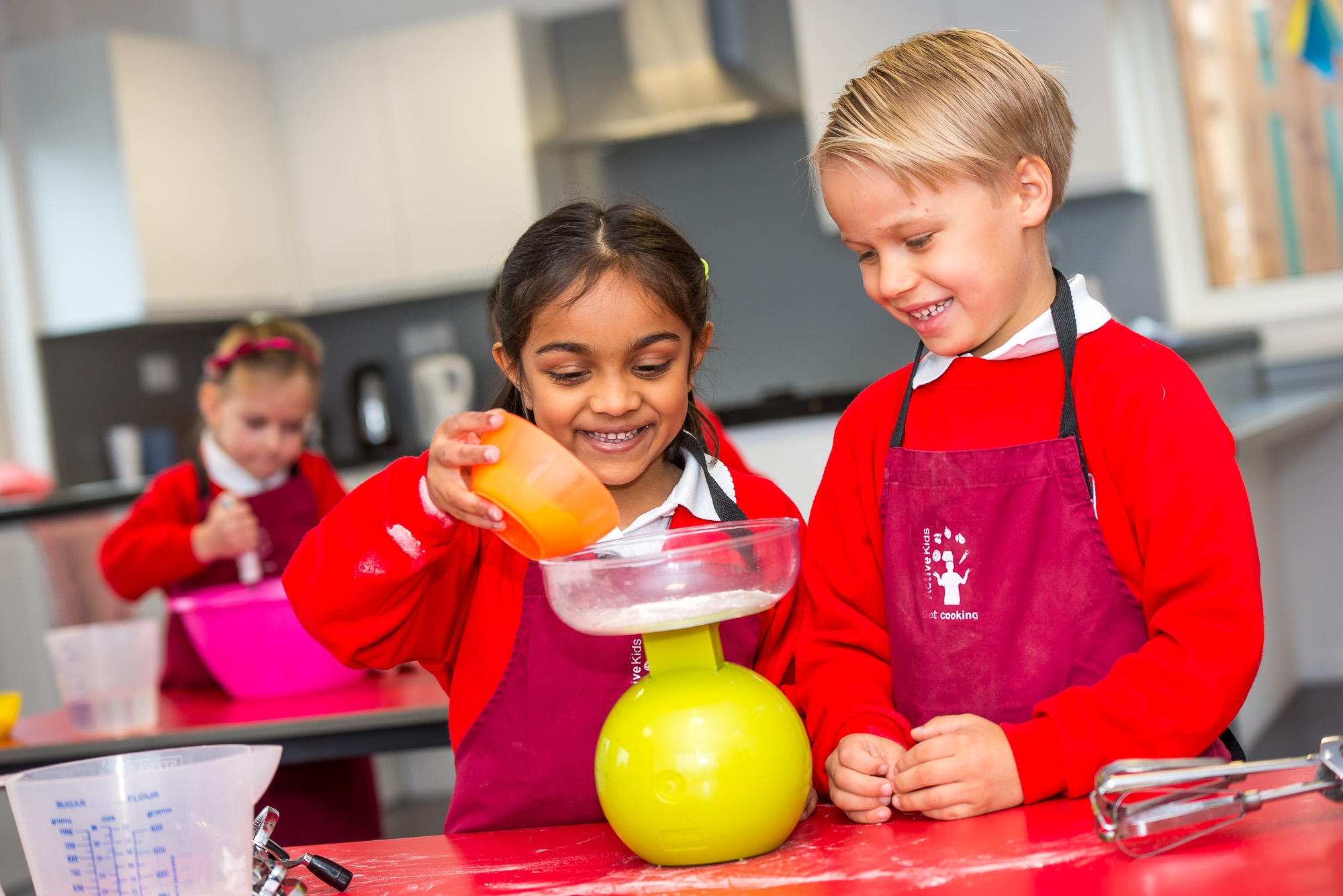# Glebe Challenge

## Glebe Challenge

Mental maths involves completing short mathematical problems without the use of a pen and paper or a calculator. It is not only useful in school, but in everyday life, such as working out change; sharing items between a group of people and working out when to leave in order to arrive at the correct time.

Mental maths can be used in place of more formal methods. For example, what is 47 + 65? It’s easier to do this in your head if you break the numbers down into their digits’ values: 40 + 60 = 100 and 7 + 5 = 12. Add those two totals and you get the answer of 112.

Improving mental maths skills is a priority of ours at Glebe. Exercising mental maths skills helps to exercise both sides of the brain and has been strongly associated with better memory skills.

To aid with exercising our mental maths skills, every week, the children undertake a Glebe Challenge. This involves the children completing a sheet of mental maths problems within a 5-minute time frame.

Each category and what they entail is detailed below:

## Copper

Number bonds to 10 and 20, including inverse

## Bronze

2, 5 and 10 times tables including inverse and related division facts

## Silver

Fractions: ½, 1/3, ¼ and ¾

1-12 times tables including inverse and related division facts

## Gold

Fractions of amounts (numerator of 1)

Fractions of amounts (mixed numerators)

Simple equivalent fractions

Decimals

Percentages

Square roots

X and ÷ by 10, 100 and 1,000 of decimals

X 9 and x 99

## Platinum

Cube roots

% of amounts

Multiplying fractions by whole numbers

Dividing decimals

Power of 4

Conversion of units

Dividing fractions by whole numbers

Fractions x fractions

Multiplying by 0.1, 0.01 and 0.001

BODMAS